# SSAT Upper Level Math : SSAT Upper Level Quantitative (Math)

## Example Questions

### Example Question #5 : Outcomes

Three fair dice are rolled. What is the probability that none of them will show a 5 or a 6?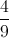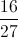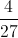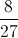Explanation:

The equivalent question is this: What is the probability that all three dice will show 1, 2, 3, or 4?

The probability that one fair die will show a 1, 2, 3, or 4 is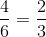, so the probability that all three will show 1, 2, 3, or 4 is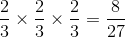.

### Example Question #6 : Outcomes

The threes from one standard deck of playing cards are added to another deck, and a card is drawn at random from that second, modified deck. What is the probability that that card will be an ace, a two, or a three?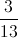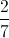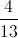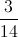Explanation:

If four more threes are added to a deck of fifty-two playing cards, then the deck will have fifty-six playing cards, including four aces, four twos, and eight threes. This makes sixteen ways to draw an ace, a two, or a three out of a possible fifty-six draws, or a probability of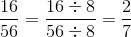.

### Example Question #7 : Outcomes

Ten balls - five green, three blue, two yellow - are placed in a hat. A ball is drawn, then replaced; then, a ball is drawn again. What is the probability that a blue ball will be drawn at least once?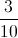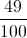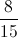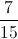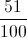Explanation:

This can more easily be solved by finding the probability that neither draw will result in a blue ball, then subtracting it from 1.

The probability that the first ball will not be blue will be seven (balls that are not blue) out of ten (balls total), or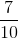. Since the ball is replaced, this is also the probability that the second will not be blue. Therefore, the probability that neither will be blue is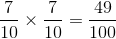The probability that at least one draw will result in a blue ball is therefore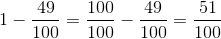.

### Example Question #8 : Outcomes

Two fair dice are rolled. What is the probability that at least one will show a 5 or a 6?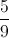Explanation:

The best way to find this probability is to find the probability that both will show a 1, 2, 3, or 4, and subtract that probability from 1.

The probability that one fair die will show a 1, 2, 3, or 4 is, so the probability that both will show 1, 2, 3, or 4 is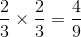.

The probability that at least one die will show a 5 or a 6 is therefore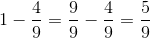.

### Example Question #9 : Outcomes

The tens from one standard deck of playing cards are added to another deck, and a card is drawn at random from that second, modified deck. What is the probability that that card will be an ace, a two, or a three?Explanation:

If four more tens are added to a deck of fifty-two playing cards, then the deck will have fifty-six playing cards, including four aces, four twos, and four threes. This makes twelve ways to draw an ace, a two, or a three out of a possible fifty-six draws, or a probability of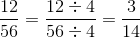.

### Example Question #10 : Outcomes

A card is drawn at random from a standard 52-card deck. The person drawing wins if the card he draws is a club, a king, or a queen. What are the odds against drawing a winning card?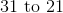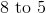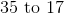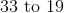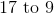Explanation:

There are 19 winning cards: the 13 clubs, the other 3 queens, and the other 3 kings (the queen and king of clubs are already counted). There are subsequently 33 losing cards, so the odds against a winning card are the number of losing cards, 33, to the number of winning cards, 19. This cannot be reduced, so the correct choice is 33 to 19.

### Example Question #1661 : Ssat Upper Level Quantitative (Math)

A card is drawn at random from a standard 52-card deck. The person drawing wins if the card he draws is a two, a three, or a black four. What are the odds against drawing a winning card?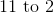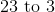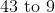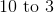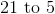Explanation:

There are 10 winning cards: the 4 twos, the 4 threes, and the 2 black fours. There are subsequently 42 losing cards, so the odds against a winning card are the number of losing cards, 42, to the number of winning cards, 10. This can be reduced to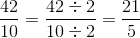or 21 to 5.

### Example Question #1662 : Ssat Upper Level Quantitative (Math)

A card is drawn at random from a standard 52-card deck. The person drawing wins if the card she draws is a red four or a black nine. What are the odds against drawing a winning card?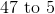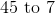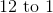Explanation:

There are 4 winning cards: the 2 red fours and the 2 black nines. There are subsequently 48 losing cards, so the odds against a winning card are the number of losing cards, 48, to the number of winning cards, 4. This can be reduced to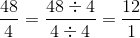or 12 to 1.

### Example Question #1663 : Ssat Upper Level Quantitative (Math)

John participates in a charity fundraiser in which he pays $1 to draw a card from a standard 52-card deck. If he draws the ace of spades, he wins$25; if he draws any other ace, he wins $5; if he draws any other card, he does not win. To the nearest cent, what is the expected value of the game to John? Possible Answers: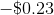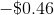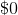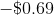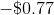Correct answer:Explanation: Since there is only one card out of 52 (ace of spades) that wins John the$25 prize, the probability of this happening is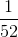. The value of this outcome to John is $24 - the$25 prize minus the $1 he paid to play. Since there are three cards out of 52 (ace of clubs, ace of diamonds, ace of hearts) that win John a$5 prize, the probability of this happening is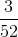. The value of this outcome to John is $4 - the$5 prize minus the $1 he paid to play. Since there are 48 out of 52 cards that do not win John a prize, the probability of this happening is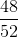. The value of this outcome to John is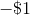, since he had to pay$1 to play.

To find an expected value of a game, multiply the probability of each outcome by its value, then add the products. The expected value of this game to John is therefore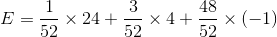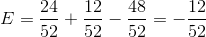dollars, or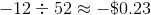The expected value of the game to Johnny rounds to.

### Example Question #1664 : Ssat Upper Level Quantitative (Math)

Sharon participates in a charity fundraiser in which she pays $2 to draw a card from a deck of cards; the deck is a standard 52-card deck plus the joker. If she draws the joker, she wins$25; if she draws the ace of spades, she wins $10; if she draws any other ace, she wins$7; if she draws any other card, she does not win. To the nearest cent, what is the expected value of the game to Sharon?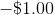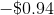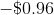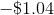Explanation:

Since there is only one card out of 53 (joker) that wins Sharon the $25 prize, the probability of this happening is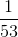. The value of this outcome to Sharon is$23 - the $25 prize minus the$2 she paid to play.

Since there is only one card out of 53 (ace of spades) that wins Sharon the $10 prize, the probability of this happening is. The value of this outcome to Sharon is$8 - the $10 prize minus the$2 she paid to play.

Since there are three cards out of 53 (ace of clubs, ace of diamonds, ace of hearts) that win Sharon a $7 prize, the probability of this happening is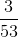. The value of this outcome to Sharon is$5 - the $7 prize minus the$2 she paid to play.

Since there are 48 out of 53 cards that do not win Sharon a prize, the probability of this happening is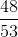. The value of this outcome to Sharon is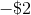, since he had to pay \$2 to play.

To find an expected value of a game, multiply the probability of each outcome by its value, then add the products. The expected value of this game to Sharon is therefore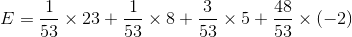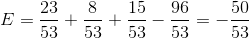dollars, or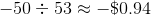The expected value of the game to Sharon rounds to.

### All SSAT Upper Level Math Resources